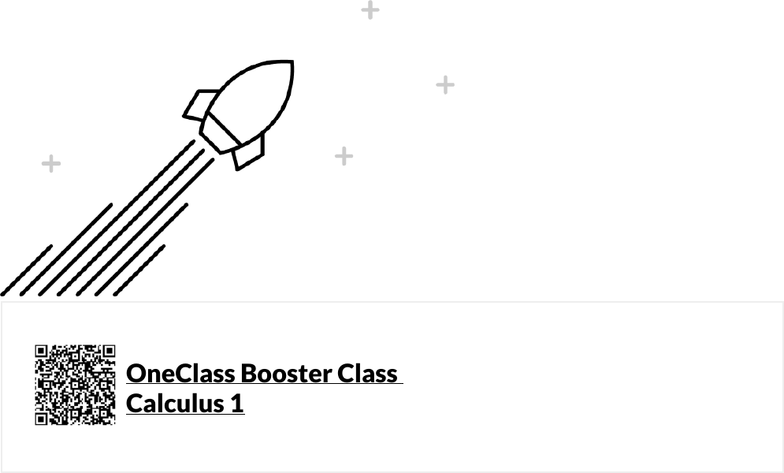# Calculus 1 Fall 2020 Final Exam Study Guide

67 views327 pagesF A L L 2 0 2 0
CALCULUS1
S T U D Y G U I D E
ONECLASSUNIVERSITY
Unlock document

This preview shows pages 1-3 of the document.
Unlock all 327 pages and 3 million more documents.1
6
17
19
23
28
35
37
This Study Guide was made using:
OneClass Booster Class
Calculus 1
oneclass.com/courses/mathematics/calculus-1.en.html
Contents
1 Represent Functions
Theory
Quiz
2 Combine Functions
Theory
Quiz
3 Transform Functions
Theory
Quiz
4 Evaluate Logarithmic Functions
Theory
Quiz
Unlock document

This preview shows pages 1-3 of the document.
Unlock all 327 pages and 3 million more documents.

41
43
59
61
65
66
69
71
74
77
80
81
85
86
90
92
96
98
103
104
108
110
5 Sketch Continuous And Differentiable Functions
Theory
Quiz
6 Determine Continuity Of Functions
Theory
Quiz
7 Apply The Intermediate Value Theorem
Theory
Quiz
8 Find Tangent Line Equations
Theory
Quiz
9 Apply Differentiation Rules Part 1
Theory
Quiz
10 Find The Derivative Of The Function
Theory
Quiz
11 Evaluate Exponential Functions
Theory
Quiz
12 Evaluate Trigonometric Functions
Theory
Quiz
13 Interpret The Meaning Of The Derivative
Theory
Quiz
14 Calculate The Average Rate Of Change And Instantaneous Rate Of Change
Theory
Quiz
15 Use Implicit Differentiation
Theory
Quiz
Unlock document

This preview shows pages 1-3 of the document.
Unlock all 327 pages and 3 million more documents.

113
114
118
119
122
124
128
130
134
138
147
149
152
153
156
157
160
165
174
176
179
184
16 Find The Derivatives Of Inverse Trigonometric Functions
Theory
Quiz
17 Use Logarithmic Differentiation
Theory
Quiz
18 Apply Rolle's Theorem
Theory
Quiz
19 Classify Functions
Theory
Quiz
20 Sketch Functions Part 2
Theory
Quiz
21 Apply Differentiation Rules Part 2
Theory
Quiz
22 Solve Related Rates
Theory
Quiz
23 Solve Exponential Growth And Decay Problems
Theory
Quiz
24 Find Minimum And Maximum Values Of A Function
Theory
Quiz
25 Determine Differentiability Of Functions
Theory
Quiz
26 Sketch Functions Part 1
Theory
Quiz
Unlock document

This preview shows pages 1-3 of the document.
Unlock all 327 pages and 3 million more documents.

192
193
197
203
212
217
222
226
229
230
234
244
245
248
248
257
258
259
261
262
263
265
266
267
268
270
271
272
273
274
281
283
284
286
27 Apply The Mean Value Theorem
Theory
Quiz
28 Graph Using First And Second Derivatives
Theory
Quiz
29 Solve Function Word Problems
Theory
Quiz
30 Solve For Limits
Theory
Quiz
31 Solve Optimization Problems
Theory
Quiz
32 Quiz Solutions
Skill 1: Represent functions
Skill 2: Combine functions
Skill 3: Transform functions
Skill 4: Evaluate logarithmic functions
Skill 5: Sketch continuous and differentiable functions
Skill 6: Determine continuity of functions
Skill 7: Apply the Intermediate Value Theorem
Skill 8: Find tangent line equations
Skill 9: Apply differentiation rules part 1
Skill 10: Find the derivative of the function
Skill 11: Evaluate exponential functions
Skill 12: Evaluate trigonometric functions
Skill 13: Interpret the meaning of the derivative
Skill 14: Calculate the average rate of change and instantaneous rate of change
Skill 15: Use implicit differentiation
Skill 16: Find the derivatives of inverse trigonometric functions
Skill 17: Use logarithmic differentiation
Skill 18: Apply Rolle's Theorem
Skill 19: Classify functions
Skill 20: Sketch functions part 2
Skill 21: Apply differentiation rules part 2
Skill 22: Solve related rates
Skill 23: Solve exponential growth and decay problems
Skill 24: Find minimum and maximum values of a function
Unlock document

This preview shows pages 1-3 of the document.
Unlock all 327 pages and 3 million more documents.

291
292
296
297
303
305
307
Skill 25: Determine differentiability of functions
Skill 26: Sketch functions part 1
Skill 27: Apply the Mean Value Theorem
Skill 28: Graph using first and second derivatives
Skill 29: Solve function word problems
Skill 30: Solve for limits
Skill 31: Solve optimization problems
Unlock document

This preview shows pages 1-3 of the document.
Unlock all 327 pages and 3 million more documents.